Reach Us+32-10-28-02-25
About New Internal Symmetries of the Integer Lattice (Z<sup>N</sup>) | OMICS International
Journal of Physical Mathematics
All submissions of the EM system will be redirected to Online Manuscript Submission System. Authors are requested to submit articles directly to Online Manuscript Submission System of respective journal.

# About New Internal Symmetries of the Integer Lattice (ZN)

Kornyushkin A*

Independent Researcher, Russia

*Corresponding Author:
Kornyushkin A
Independent Researcher, Russia
Tel: 02541842138
E-mail: [email protected]

Received date: May 07, 2016; Accepted date: May 24, 2016; Published date: June 01, 2016

Citation: Kornyushkin A (2016) About New Internal Symmetries of the Integer Lattice (ZN). J Phys Math 7: 178. doi:10.4172/2090-0902.1000178

Copyright: © 2016 Kornyushkin A. This is an open-access article distributed under the terms of the Creative Commons Attribution License, which permits unrestricted use, distribution, and reproduction in any medium, provided the original author and source are credited.

Visit for more related articles at Journal of Physical Mathematics

#### Abstract

The numerous internal symmetries are found in N-dimensional integer lattices (ZN). The relation of these symmetries with the new mathematical category named the Masks (or Neighborhoods) is shown. A set of definitions for the Correct Masks and Perfect Masks is presented; the identity between the Correct and Perfect Masks is hypothesized. The relationship between the Perfection of the Mask and the new category named “Mathematical String” is shown. The Correctness of the several Masks in ZN (N=1,2) is proven and a simple method to find the Correctness for all other N is outlined. The hypothesis of high population density of Perfect Masks in integer lattices ZN with large N is stated.

#### Keywords

Symmetries; Integer lattice

#### Introduction

A new set of internal symmetries for N-dimensional integer lattices ZN are inseparably linked to a new mathematical category named the Mask (Neighborhood). This category represents a successful attempt to generalize the notion of integer number (Z). There exists a generally accepted concept of hierarchy of numbers: Boolean number ⇒ natural number ⇒ integer number ⇒ real number ⇒ complex number. The author suggests a slightly different classification: the “tree” forks starting with the integer numbers. After the integer numbers, the standard limb (where all “continuous” numbers belong) goes to the right, and a discrete-space generalization of the “integer number”, which is referred in this paper as the Neighborhood (Mask), goes to the left. As the natural numbers can be prime or composite, so the Neighborhoods can be Correct (or Perfect) and... “not quite Correct”, i.e., Incorrect. For example: Neighborhood {-5;-2;-1;1;2:4} in one dimension is Correct and (apparently) Perfect, yet nothing can be said, for instance, about Neighborhood {-5;-3;-1;1;2:4}. The notion of Correctness, as we.

In the beginning, consider replacing the term Neighborhood with its synonym, the Mask. The reasons of doing this will be discussed below.

Let us define Mask M in N-dimensional space as the set of n integer, nonzero, and the different vectors of dimension N: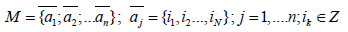The Mask is normal if among other vectors it comprises all the vectors (we can call it unit vectors) wherein one element ik (k=1,..., N) is +1 or -1 while all others elements are 0. The Mask is called primitive if it contains ONLY unit vectors. Thus, primitive Masks are a subclass of normal Masks. The Mask that for any vector { i1,i2,…, iN} contains vector { -i1,-i2, …, -iN} is called central- symmetric. In one-dimensional integer space, any Mask is easy to describe with two numbers (m, k) where the binary representation of m gives negative i, and the binary representation of k (after mirroring) gives positive i. For instance, (19,11) stands for Mask {-5;-2;-1;1;2;4}. This is due to the fact that (19,11)=(24+21+20, 20+21+23)=(10011; 1101); after inversing (“mirroring”) the sequence of digits in the second component (1101Mir ⇒ 1011), we obtain {-5;- 2;-1;1;3;4}. Similarly, expression (21,11)=(24+22+20, 2M0+21+23)=(10101; 1101 Mir)=(10101; 1011) denotes Mask {-5;-3;-1;1;2;4}. In the future, we will consider only normal Masks. From this point, any Mask=normal explained in Figure 1.

#### Definition of the Correct Mask. Theorem 1

Consider lattice ZN. Some or none of lattice dimensions can be closed (like in a torus) whereas all the other stay infinite, this property has no impact on our future analysis. Take any Mask in N-dimensional integer lattice and add Zero vector to it (all the elements of Zero vector equal 0). Using this Mask with Zero vectors we define a Cellular Automaton (CA; further we refer to this Automaton as Table CA) with the following properties:

1). CA cells can be in one of 6 states. At every moment of time, the state is one of the letters from following set

{-x;-y;-z; +x; +y; +z}

2). The state of each cell in the next moment of time is defined by Transition Tables R-x, R-y, R-z, R+x, R+y, R+z: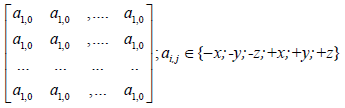The number of columns in the Tables is n (the number points in the Mask) plus 1. Then we take C as the number of rows in the Tables. Both n and C will be constantly used in the text below. Example of Transitional Table for a Mask (1,1); n=2, C=9 (the rule how to find C for a particular Mask will be explained further on):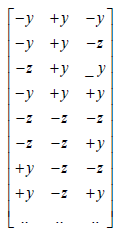And similarly for the other letters. We remind here that there are +1 columns in Transition Tables R due to inclusion of Zero vector. No two rows of the Transition Tables can be the same.Then a set of standard routines is executed. First, we arbitrarily enumerate our Mask points plus Zero vector (the center) with numbers j. Figure 2 shows Zero “point” with a dot). Secondly, we superimpose (overlay) each cell in ZN with zero point of our Mask. Note: it is due to that “overlay” operation, the name “Mask” seems more appropriate than the “Neighborhood”.

Figure 2: The illustration of one step of the Table CA “run” for a primitive Mask in two dimensions. For clarity, the Automaton run is shown for a single cell.

Thirdly, we write out row {a0, …, aj, …, an} containing the sequence of states that are taken from the cells on the lattice ZN where the enumerated vectors comprising our Mask end up illustrated in Figure 2. Fourthly, we check Transition Tables R-x, R-y, R-z, R+x, R+y, R+z on a subject of whether or not such a row exists in any of the Transition Tables. If we find such a Table, we put its index as the state of CA in the next moment of time (t+1). For example, if row{ a0, …, aj, …, an } appears in table R-x, then the next state is -x; if some other row appears in table R-y, then the next state is -y, etc. Because no rows of the Transition Tables can be the same, there is only one state of lattice ZN in each time point. Figure 2 shows the transformation of arbitrary selected element from integer lattice Z2. It illustrates a single step of operation of a Table CA for a primitive Mask in two dimensions on a closed lattice (i.e., the lattice without “boundary”) in this particular case, a surface of 6 x 6 torus. In accordance to CA definition, the same operation should be performed at once for all cells of CA Figure 3. Let us compare, for example, the run of our Cellular Automaton with that of well-known CA named Rule 30 by Stephen Wolfram  (Figure 4).

Figure 4: The plot “Automaton state vs. Time” for Wolfram’s Automaton “30”.

Rule 30 has 2 states of the cells while our CA has 6. Rule 30 works or runs (if we consider the “works” through our terms) for a primitive Mask (1,1) in one dimension. The binary decomposition of 30 is abbreviation for the transition rules  and Table 1.From those rules, we can determine the Transition Tables: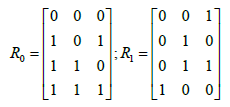(4)

 Current Pattern 111 110 101 100 011 010 001 000 New state for center cell 0 0 0 1 1 1 1 0

Table1: Transitionrules for Rule30 Automaton.

We can notice that contrary to our Automaton, CA Rule 30 is not reversible (see explanations below). Any Transition Table has a dedicated column (numbered as “h”) which is associated with the Zero Vector that we added to the Mask. It is logical to assume h=0 for the dimensions greater than one. Yet, in the case of one dimension, it is more convenient to leave the "natural” order (for instance, h=1 for Automaton Rule 30).

3) The obtained Transition Tables are interdependent and can be obtained from table R-x after following substitutions:

R-x: e={-x,-y,-z,+x,+y,+z};R-y:e1={-z,-x,-y,+z,+x,+y};R-z: e2={-y,-z,- x,+y,+z,+x}; (5)

R+x: e={+x,+z,+y, -x,-z- y,};R+y:e4={,+y,+x+z, -y,-x, z,};R+z: e5={z,+y,+x,,-z, -y, -x }

These substitutions form a group; its multiplication table (u x v) is shown in Figure 5.

4) Our Automaton is reversible. The inverting Transition Tables are calculated from the same Table.

R-x using following substitutions: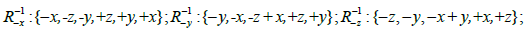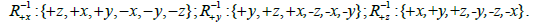5) When getting started from any initial state of the integer lattice (ZN) filled with only -x and +x (further we refer to this the state as the Beginning Point or BP), our CA will last forever. In other words, all the time as CA runs, only those states appear which are present in tables R.

6) At least one row containing only letters “-x” and “+x” must be found in tables R-y or R-x or Rz

or R+z. (In other words, the “unionized” table R-y U R+y U R-z U R+z should contain this row).

7) All points (vectors) in the Mask are important. In other words, no point of the Mask can be safely eliminated without changing the Automaton.

If, for a given Mask, it is possible to find CA meeting all seven conditions, then we call this Mask Correct. If it is not possible (or until proven otherwise), we call such a Mask Incorrect. Let us explain why the very existence of Correct Masks is a “miracle” of its kind. It is far from being obvious that at least one Mask is Correct in some N-dimensional space. From point 5) it follows that the Automaton must be able to make at least the first step, therefore, the Tables should contain all the 2n+1 states formed from the letters of the same type “x”. At some initial conditions after the first step (see point 6) there must appear a new letter (not “+x” or “–x”) in the states. Now, we are curious about the way the rows containing these letters are transformed. Assuming that we know the answer, it will immediately be transferred into six Transition Tables by performing substitutions, see expressions (5). Note that it will also be transferred into six Inverting Tables and, in the end, into the same Transition Tables. Each step causes new transfers. It may very soon turn out that two identical rows appear in two different tables, and this is prohibited. Yet, a situation is not that bad. As a matter of fact, there is a huge number of the Correct Masks and apparently in all dimensions.

Figure 6:In the entire figure, black colour means that the Mask is Incorrect. Panel A shows the Correct Masks (m, k) in one dimension - those are the cells with the numbers inside, colored differently from black. Because the number of rows in Tables R is always odd, we introduce important parameter: C1/2=(C-1)/2, which value is indicated in the cell. Numbers in cells: on the top – the number of points in a Mask; on the bottom – value C1/2for the same Correct Mask; a question mark means that C1/2was not calculated, yet most likely, the Mask is Correct. Values of ? represent the “density” of our Table CA for seven Masks. Bordered area to the left shows ? calculated for the cells indicated by arrows. Panel B shows C1/2 for the first Correct Masks in two dimensions. We remind that in this case “n” equals the number of cells in the Mask minus one whereas value C1/2 will be explained in the next chapter where we will deal with the Transition Tables. Further details are discussed in the text.

Proof: Note, the proof for Theorem 1 is obtained using personal computer. The software program with the source code (common to dimensions N=1 and N=2). In the beginning, we present the Transition Tables for primitive Masks in one (Figure 7A and 7B) dimensions as well as for Mask (3,1) in one dimension (Figure 7C). Let us explain what is depicted in Figure 7. In each of three panels, we move from the left to the right. Some rows in Figure 7 containing only letter “y” and marked with inclined lines (shading) indicate that these rows are important to perform the “mathematical induction” step in our proof, which is conducted further on in the text. Let us consider letter “y” (note that in case of letter “x” everything is considered similarly). To prove the Correctness of our Mask, first, we need to make sure that our table contains all the 2n+1 rows consisting of one and the same letter“x”. The R-x table has a row containing only “-z”. Therefore, table R-z has a row containing only “-y” (see point 4), and table R+x has a row containing only “+y”. The remaining (2n+1-2)/2 variants are distributed over tables R -x and R+z. Moreover, the variants in R-x are complementary to variants R+z (note that all rows from R-x are multiplied by -1 in R+z). Let us check the number of shaded rows. Consider panel A (Figure 7): on one hand, we have (22+1-2 )/2=3 rows containing only letter “y”. On the other hand, if in the same panel A, we sum up the numbers in the column S for rows containing only “y” (S stands for symmetry, explained below) then 1+2=3. Panel B (Figure 7): similarly, using the above expression we can find (23+1-2)/2=7 rows or, on another hand, summing up number in columns S, we get the same number 7 (S=1 everywhere because of no symmetry for the Mask). Finally, panel C: (24+1-2 )/2=15 or summing up the rows with account of their symmetry we get 1+4+4+2+4=15 lines.

Figure 7:Tables R-x for Correct Masks. Panel A for Mask (1,1); panel B for primitive Mask in two dimensions; panel C for Mask (3,1) in one dimension. The explanation is in the text.

Column “Δ” is just a sign of a letter in “zero” point (column) of table R (the relationship is shown by the connected dots; Figure 7). More detailed information about that column will be given in the next chapter. Column “Npr (Figure 7C) shows the number of instances the given configuration appeared in our proof (see below). The values in this column are given in multiples of C (i.e., this entire column should be multiplied by 81). Then for Figure.7A and 7B it turns out that this entire column is filled only with value 1. We could have hypothesized that all the values in column Nprwere multiples of C all the time. But this is not so. This hypothesis turns invalid already for the next Correct Mask (5,3). Column “S” serves to reduce the list of entries. Each row can be found S times in that list, taking into account the axial symmetry (Figure 7A) or square symmetry (Figure 7B).

Let us return to the proof. If table R-x is already known for some Mask, then the following action on the proof is clear. Yet, we are not certain about the outcome of such a process.

We made the first step in the mathematical induction, i.e., we showed that all 2n+1 rows containing only letter “x” are present in the Transition Tables. Now, let us move on. Given: assume that at time point t in every point of CA the content of the Mask is permitted, i.e. all the rows in the Masks belong to tables R. It is necessary to prove that at the next time point (t+1) our CA will have the same property. The solution can be found through the proof by exhaustion performed on a personal computer.

Let us unite all Transitions Tables (R-x, R-y, R-z, R+x, R+y, R+z) into one Table and named it R(6). It has 6C rows numbered 0, 1,… k,… 6C-1 and the numbering of its rows has the following construction. First, the rows of table R-x are placed in a descending order in accordance with the selected seniority - x>-y>-z>+x>+y>+z. Other Transition Tables can be found by using corresponding substitutions, i.e., using the rule (5) from point 3, and numbering in these Tables does not change relatively to that in R-x. After that, the Tables are concatenated (here it means the second one is attached to the bottom of the first one, etc) in similar order: R-x … , R-y … R-z … R+x … R+y … R+z. To determine the specific Table for the row with number k, one has to determine the floor function [k/C], where C is the number of rows in a single Table of our Mask.

Let us introduce a new term. Let us name Mkj as the Mask filled with the row numbered kj from R(6); kj=0, …, 6C-1 (see an example of such a filling in Figure 8). We call set {k0, k1,, …kn} from n+1 (nnumber of the points in the Mask) filled Masks “illegal” if after the placement of set Mk0 into a point with number 0 of our Masks, Mk1 - into a point with number of 1 our Mask, etc., some point of our lattice will be filled with two different letters, that is the letters not equal to each other. Otherwise we call our set “legal”. Now consider, for example, the primitive Mask in two dimensions (see panel B in Figure 7). In Figure 7 (panel B), a natural sequence of row numbering in the considered Transition Table R(6) is truncated (or skipped) in many places due to symmetries (e.g., instead of writing in three rows, one after another, we have placed a single row and indicated the corresponding symmetry). Yet, if we write out all the rows of combined Transition Table R(6) as they are, then it will turn out, that for instance, rows number k0=220; k1=165; k2=221; k3=358; k4=177 form a “legal” set (Figure 8). It is clear that only a limited number of all sets are “legal”. For example, it is easy to see, that if we have any “legal” combination {k0, k1, …, kn} and h=0 (Figure 7 to determine h) then any set {m, k1,…, kn} where m it is not equal k0, will be“illegal”. Further we find “legal” sets {k0 k1 …, kn} using the n+1 nested loops (each loop considers 6C variants for ki) thus going through all possible combinations of ki (Figure 9).

Figure 8: “Legal” set {220, 165, 221, 358,177} in case of a primitive Mask in two dimensions.

Figure 9: Five nested loops in the proof of Correctness of a primitive Mask in two dimensions. In each loops, it is checked: i) whether the row {i0, i1, i2, i3, i4} belongs R(6); and ii) whether this row belongs to R-1J, where J – the value of the Mask ke (k0) in a zero point in time t. In case of Figure8, row {-z,-z,-z, y,-z} belongs to R-x and to R-1+x.

For each “legal” combination, we determine a row in the Mask in consecutive point in time {[k0/C], [k1/C],…,[kn/C]} and make two checks: i) whether or not the row under consideration can be found in R(6) and ii) whether or not the inverse transformation works. After that, we add “one” to column Npr corresponding to that row and move on to a next step in a cycle...We reach the end, and declare that the Theorem is proved. So, we have got a key observation. If we have the Transition Tables the proof of Correctness is always leads to a success. This fact can be considered as the greatest mystery in mathematics.

#### Definition of Simple reversible Automaton for the Mask

Figure 10: Panel A – illustration of Simple CA run. One needs to take a notion that each grey “band” shown in this figure is actually a torus (it is rather difficult to depict the considered operations on a torus). Any run that starts from the Beginning Point (i.e., the state filled only “A” and “C” cells) follows the circle: the Beginning Point => the Mirror Point (MP also comprises cells “A” and “C”) => Beginning Point. We have two runs: Ordinary and Additional (in the initial conditions of the latter, the replacement A⇔C is made). Ordinary and Additional runs may have different Half Periods: T and T*. Yet, for some Masks (the Correct ones), it becomes clear that after removing all cells B from both pictures, the pictures will look the same after making replacement all picture Automaton state “vs time” for any of our runs (Ordinary or Additional). The straight yellow A⇔C. Panel B shows the w lines indicate the Beginning and Mirror Points. Orange lines connecting the centres of cells C show distinct C/B “bands” which do not cross each other. Further explanations see in the text.

Imagine a chess figure which makes only a one-unit move forward or backward along any of axes of the N-dimensional integer lattice (at each step, the figure can move along some arbitrary axis); also note that it is permitted for this figure to make a move on the field where the figure was in the past. It is obvious that the figure can eventually visit all the points of any closed integer lattice and return to its starting position. So, using the course of this figure we transform any N dimensions into one as shown in Figure 10B). After that, to prove that our bands do not cross each other bands, we use the property of a normality, .

Further, there is the main observation that, for any Correct Mask, CA of arbitrary size, and any initial conditions selected in the diagram “Automaton state vs. Time” where all B cells are removed, pictures for the “Ordinary run” and “Additional run” (wherein the replacement of the initial conditions A⇔C is made), literally coincide after the replacement all of cells A⇔C. Consider the issue again and in detail. Let us name the diagram “Automaton state vs. time” for the normal run of Automaton as the Ordinary World; and a similar diagram for the “additional run”, as Parallel World. First, let us introduce the terms describing the Ordinary World. The state of Simple CA in point r as a function of time t is denoted as

fABC(t,r)∈{A;B;C} (7)

Then, let us introduce function b which indicates whether a given cell is the B cell at a given time, or not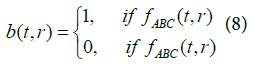Transformation functions (with B removed) are defined as follows also explained in Figure 11.

Figure 11: Illustration to finding the Transition Tables R Panels A and B: the diagrams “State of Automaton vs. Time” for two Automaton runs (for Ordinary and Parallel worlds). Panels C and D – the corresponding transformations of cells with letters “A” and “C” after removal of all cells with letters “B”.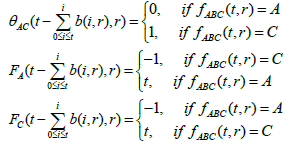Let us take the primitive Mask in one dimension on the closed row (a ring) of ten points, two points of 10 being colored with two different (“opposite”) colors. Yet, we repeat one more time, that all our methods can be applied without any changes to any Correct Mask in the N-dimensional space, for Automaton of any size, and for any initial conditions (Figure 12).

Figure 12: Illustration of the transformation step shown in Figure 11 - i.e., the transition from panel A to panel C. First, we remove letters B from the cells (empty the cells of letter B). After the removal, letters “At” and “Ct”, where t is the row number (time coordinate) in the original (t, r) space, move up to fill the vacant spaces. After that, we apply Eq. 10 to “At” and “Ct”, thus converting the “colors” into numbers, and obtain Figure 11C.

Let us introduce four new functions as follows: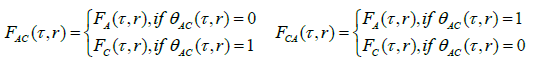Note that the construction of FAC (τ, r) was shown merely as an intermediate supplementary function to demonstrate the construction of similar function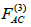with the only difference in mod 3 (we emphasize that mod 3 is an important manipulation, it makes all other things happen). It should be understood that these operations create completely different tables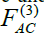and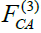. We introduce new notations: 0 ⇔-x, 1⇔ -y, 2⇔-z, 3⇔+x, 4⇔+y, 5 ⇔+z. We can see from the illustration of Figure 13 that these notations definitely give us the Transition Tables. The first Table is for one direction in time, and the second, for the opposite one. (If we have taken FCA function instead of FAC one, it would lead to replacement R⇔R-1).

Figure 13: Continuation of illustration shown in Figure 11. In this figure, panels (A, C) and (B, D) show the two pairs of practically identical Tables, the only difference between the top Table and bottom one is that in the bottom one, the numbers in the cells are replaced with letters (see straightforward conversion rules depicted on the left and right sides). Our task at this point is to find NEW rows in the table R-x. In each drawing (see Figure 12C and 12D). We selected three arbitrary points - the results of the F and FC(3)functions where the input parameter is the same cell (τ,r) in the corresponding top panels (are shown in ovals). For three points we can determine 6 new rows in different tables R (the corresponding rows are allocated in a gray three-cell rectangle). In the table R+x – a row {+y,-z,+z}; in the table R-1+x – a row{+z,+z,+z}; in the table R-x – a row {- y,+y,- y} etc. All these rows from the substitution (see Expressions 5-6) are then transferred to the table R-x. Further these rows are compared to the ones that are already present in R-x, and if the new rows aren’t there, we add those rows to the Table .

Now it is clear how to obtain Transition Tables. First, we set random initial conditions from A and C states defined on “small” integer lattices that are closed in all directions and form the torus and turn on a Simple Automaton on the Mask. Then, for every three moves of a Simple Automaton on the time scale, we can find L (total cell number) multiplied by 2 states in each of our new tables (3) AC F . Finally, we stop after we reach the point of half-cycle and analyze the result. Then we change the initial conditions and repeat the trial. At each point in table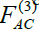,we can determine 12(!) new rows R-x taking into account the inverse motion. If the Mask is Correct, then R-x will be entirely filled in a short time. After that, it is necessary to perform standard check for Correctness (see Chapter 3).

#### Definition of a Perfect Mask. Strings

So, let us repeat again. Let us take a look on two runs (we call it as Standard and Additional) of i) a Simple Automaton on the Mask (fABC; as a function of time t) and ii) Table Automaton on the same Mask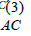; as a function of time τ) induced by Simple Automaton. The cells we have obtained are the same and numbered with integers r. In the case of Simple Automaton, our run (the Ordinary World) starts with state filled with cells containing letters “A” and “С” at time t=0; Figure 10. In the additional run (Parallel World), the replacements A⇔C take place. In the case of Table Automaton, our run (the Ordinary World) starts with state filled –x (cells with letters “A” in Simple Automaton; t=0) and +x (cells with letters “С” in Simple Automaton; t=0). In the additional run (Parallel World) of the Table Automaton the replacements -x⇔ x take place.

Let us introduce function Δ(τ,r).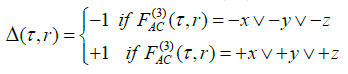And write out without any proof several formulas connecting our two Automatons. In any point r, parameters t and τ are connected by the relation: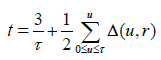Eventually, our final observation is that if all this is correct for the Ordinary World, then for Parallel Word (the functions are designated by asterisk) and for all τ и r the following is true explained in Figure 14.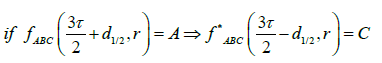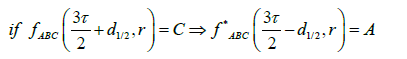If for some Correct Mask and for every run the Eqs. 13-17 are satisfied, then we can call our Mask (as well as two of its CA) Perfect. Further on, we will portray this situation as “the two CA make their runs (from BP to the MP) by a use of a contiguous String”. Note that theoretical physics has its own “strings”, but even if the author has not known about it, he would have called this object with this word. We will show our String on some simple example (Figure 15). Let us show in the same drawing each of two one-dimensional runs of Simple Automaton for Masks (3,1). The length of row (closed into a ring) is 15 cells. Initial conditions are as follows: one point of “opposite” color (in Ordinary World: all cells C, one cell is A; in Parallel World: all cells A, one cell is C). Moreover, we use line of different colors to depict C/B «bands» for both runs: the green line indicate the C/B band in the Ordinary World while the blue line shows the C/B band in the Parallel World. Horizontal strips shown in panel A of Figure 15: x (light-blue) designate t mod 3=0, band y (white) t mod 3=1, and z (pink) t mod 3=2, where t is time (see above). Note that if we had taken normal but Incorrect Mask (5,1) instead of Mask (3,1), we would have obtained no Strings at all. Our beautiful picture from Figure 15 would have turned into a messat once. Coming back to that chess figure from Section 4 which carries out converting the N dimensions of integer lattice into a single one, the author suggests (he didn’t check it, yet) that whatever the method of transformation is chosen, the String will keep the same appearance: i.e., the distance (taken at modulus) between the adjacent cells (in one measurement) will never be more than one.

Figure 15: Panel A shows the two superimposed runs for Mask (3, 1). It seems to be chaotic at the first glance. Panel B: vertical bars designate halves of Strings d1/2 (see Equation16) from the Ordinary World (pink bars) and Parallel one (green bars). It is not easy to recognize the String in the picture taken as a whole, but it is there. The situation is very unusual. Both Automatons work completely independently, yet the two runs are connected through some sort of “wormhole”.

It is possible to suggest that analogously to Theorem 1 we can also prove the Eq. 16. Yet, what to do next? Well, it is necessary to investigate algebraically our Strings in N dimensions. Obviously, it is quite a challenging task.

How many “problems” do we have? In other words, how large is the number of all Correct Masks? We introduce, informally, certain number K which is the approximate number of Masks in all dimensions, for which the Jaguar - the most powerful supercomputer on this day (February 2016) - can prove the Correctness of all the Masks in one day or maximum a week. (This definition, obviously, is necessary to correct somehow. It is well known, for example, that in one dimension, all Masks (-2k+1, 2k +1) are Correct and C (=81) for those Masks is not very large. We, of course, do not consider such Masks! We do not know how large is K. It can be equal to 10,000 or may be equal to a factorial of 100. No doubts that the Jaguar is a powerful computer. On the other hand, the number of inspections grows very rapidly with the increase of n and C. However, the inspections use the algebraic operations/steps of similar type. So perhaps it is better to do the calculations using a specialized computer.

As of today, the general program has been written to prove the Correctness of any Mask in one and two dimensions. shows the proofs of the Correctness for all of Masks from Figure 6 with n<9. The breadth of the proof at this point is limited by a performance of author’s personal computer. The Jaguar can increase “n” to several dozen (in one dimension) and number “N” to four or even five. In brief, Mathematical Strings definitely exist and in huge numbers. The author still does not know the answer to the question: whether or not all central-symmetric Masks are Correct Masks in all dimensions. The author employed the personal computer for weeks searching for abnormalities. The dimensions 1, 2, 3 have been checked. The criterion of Incorrectness was getting 2 in the expression AC AC* (Figure 11). Yet, not a single Incorrect Mask among central-symmetric ones has been found. To make the situation more intriguing, we note that there exist special Incorrect but “self- healing” Masks-Strings . We cannot exclude a situation that starting with some n and N our proof stops working. Yet, the author tends to believe that a probability of such an outcome is extremely low. We suggest that all the material presented in the above to be named as the foundation of Theory of Mathematical Strings. Note: our theory should not be confused it with a well-known theory of strings in physics.

#### Conclusion

Let us briefly summarize our findings. The Number 3 (in other words, a group from Figure 5, or configuration 2x3) generates theunimaginable number of different symmetries in the N-dimensional integer lattice ZN. Here we remind that the number of symmetries is greater than the number of central-symmetric Masks in the N-dimensional integer lattice ZN. Computer calculations indicate (yet, indirectly) that in ZN with large N almost any Mask is Correct, and each Correct Mask represents a new “symmetry” in this ZN. Theauthor developed the approach to finding the Correct Masks for lowdimensional ZN. Utilizing the same approach (yet exploiting capabilities of a very powerful computer) it is feasible to find the Correct Masks for integer lattices of higher dimensions. Our main idea is illustratedby the following figure. It seems certain that these symmetries are directly related to the physical nature of space. We do this to suggest that professionals in the field of theory of numbers should take over the theoretical physicists concerning the question of constructing a consistent theory of the Universe. Yet, while physicists argue and debate about various peculiarities of string theory, one can contemplate how to use the theory of Mathematical Strings to build a consistent model describing fundamental properties of space (Figure 16).

Figure 16: In fact, any N-dimensional lattice by action of numerous Strings turns into N- dimensional (“latticed”) sphere at large N.

#### Acknowledgements

The author thanks Dr. Eugene Moskovets for reading and correcting this manuscript.

#### References

Select your language of interest to view the total content in your interested language

### Article Usage

• Total views: 9162
• [From(publication date):
June-2016 - Nov 15, 2019]
• Breakdown by view type
• HTML page views : 8988Can't read the image? click here to refresh
###### Peer Reviewed Journals

Make the best use of Scientific Research and information from our 700 + peer reviewed, Open Access Journals

### Clinical & Medical Journals

International Conferences 2019-20

Meet Inspiring Speakers and Experts at our 3000+ Global Annual Meetings

Top